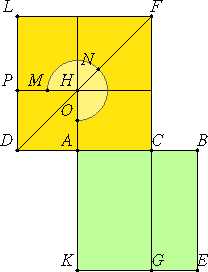# Proposition 1

If a straight line is cut in extreme and mean ratio, then the square on the greater segment added to the half of the whole is five times the square on the half.

Let the straight line AB be cut in extreme and mean ratio at the point C, and let AC be the greater segment. Produce the straight line AD in a straight line with CA, and make AD half of AB.I say that the square on CD is five times the square on AD.

I.46

Describe the squares AE and DF on AB and DC, draw the figure in DF, and carry FC through to G.

Now, since AB is cut in extreme and mean ratio at C, therefore the rectangle AB by BC equals the square on AC. And CE is the rectangle AB by BC, and FH is the square on AC, therefore CE equals FH.

And, since BA is double AD, while BA equals KA, and AD equals AH, therefore KA is also double AH.

VI.1

But KA is to AH as CK is to CH, therefore CK is double CH. But the sum of LH and HC is also double CH. Therefore KC equals the sum of LH and HC.

But CE was also proved equal to HF, therefore the whole square AE equals the gnomon MNO.

And, since BA is double AD, therefore the square on BA is quadruple the square on AD, that is, AE is quadruple DH.

But AE equals the gnomon MNO, therefore the gnomon MNO is also quadruple AP. Therefore the whole DF is five times AP.

And DF is the square on DC, and AP the square on DP, therefore the square on CD is five times the square on DA.

Therefore, if a straight line is cut in extreme and mean ratio, then the square on the greater segment added to the half of the whole is five times the square on the half.

Q.E.D.

## Guide

#### Use of this theorem

This proposition is used in the proofs of XIII.6 and XIII.11. Those propositions are in turn used to make conclusions about the sides of the icosahedron and dodecahedron constructed in propositions XIII.16 and XIII.17.